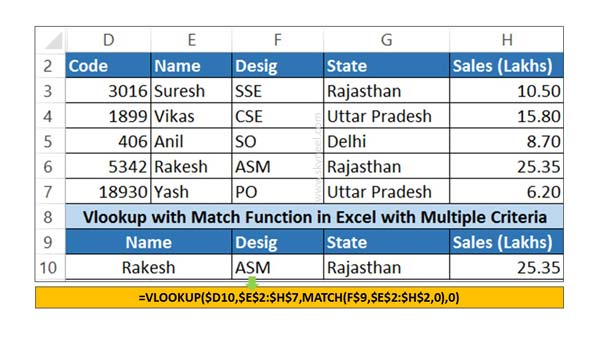# How to use VLookup Match function in Excel

VLookup Match located Lookup & reference category it's important & advance function which allow to perform a matrix lookup than normal lookup

VLookup Match one of the most powerful and important function in Excel. Sometime we need to use VLookup and Match function together to lookup specific value. If you need better control over during lookup and match the value then you must have to know how to use VLookup Match function in Excel.

### How to use VLookup Match function in Excel

This function allows you to reduce the chances of error and improve the capability of VLookup function.

During searching any specific value, you can view any error when any kind of changes are done in your workbook. If you delete or add any column in table array then you can get either an incorrect result or an error message. The main reason is that the column number is static and it doesn’t change when any column delete or add.

### VLookup function in Excel

Microsoft Excel VLookup function is used to search specific value in the extreme left column of the table_array. This function provide the value in the same row which is based on the index number.

VLookup (lookup_value, table_array, col_index_num, [range])

Lookup value is used to search in the extreme first column of the table array.

Table array is Two or more columns of data.  You can set any cell reference of a range or range name.

Col index num is the column index_number in the table from which the searched value must be returned.

Range_lookup: It is an options part of this function. There are two options available in this part.

TRUE: Appropriate Match: This option work properly when first column of your table will be sorted in ascending order only otherwise it displays wrong result.

False: Exact Match: VLookup by default check only on exact match method.

### Match Function in Excel

This function returns relative position of an item in the given array or range of cells, which match a specific value in specific order.

MATCH (lookup value, lookup array, [match_type] )

lookup value is the value which you want to match in lookup_array. The lookup_value argument can be any number, text, logical value or a cell reference to a number, text, or logical value.

lookup array is the range of cells used for search.

match_type: It is an optional. The number -1, 0, or 1. The match_type argument specifies how Excel matches lookup_value with values in lookup_array. By default value for this argument is 1.

## Steps to use VLookup Match function in Excel

If you want to use VLookup Match function together in Excel, then you must have to follow given simple steps, have a look.Step 1: Create the following structure to understand how to use VLookup Match function in Excel.

Step 2: To search desig, state and sales of any particular employee at once then you have to apply following given VLookup Match function in cell F10

=VLOOKUP(\$D10,\$E\$2:\$H\$7,MATCH(F\$9,\$E\$2:\$H\$2,0),0).

Step 3: Now, copy cell F10 function in G10 and H10 to get the state and sales amount of lookup employee.

I hope after reading this guide you can easily use VLookup Match function in Excel. If you have any suggestion regarding this guide then please let me know. Thanks to all.